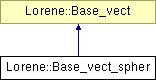# Lorene::Base_vect_spher Class Reference [Tensorial fields]

Spherical orthonormal vectorial bases (triads). More...

`#include <base_vect.h>`

Inheritance diagram for Lorene::Base_vect_spher:List of all members.

## Public Member Functions

Base_vect_spher (double xa0, double ya0, double za0, double rot_phi_i)
Standard constructor.
Base_vect_spher (double xa0, double ya0, double za0, double rot_phi_i, const char *name_i)
Standard constructor with name.
Base_vect_spher (const Base_vect_spher &)
Copy constructor.
virtual ~Base_vect_spher ()
Destructor.
void operator= (const Base_vect_spher &)
Assignment to another `Base_vect_spher`.
void set_ori (double xa0, double ya0, double za0)
Sets a new origin.
void set_rot_phi (double rot_phi_i)
Sets a new value to the angle `rot_phi` between the x --axis and the absolute frame X --axis.
virtual bool operator== (const Base_vect &) const
Comparison operator (egality).
virtual void change_basis (Tenseur &) const
Change the basis in which the components of a tensor are expressed.
virtual int identify () const
Returns a number to identify the sub-classe of `Base_vect` the object belongs to.
virtual void sauve (FILE *) const
Save in a file.
void set_name (const char *name_i)
Sets the basis name.
const char * get_name () const
Returns the basis name.
virtual bool operator== (const Base_vect &) const =0
Comparison operator (egality).
bool operator!= (const Base_vect &) const
Comparison operator (difference).

## Static Public Member Functions

static Base_vectbvect_from_file (FILE *)
Construction of a vectorial basis from a file (see `sauve(FILE* )` ).

## Protected Member Functions

Base_vect_spher (FILE *)
Constructor from a file.
virtual ostream & operator>> (ostream &) const
Operator >>.

## Protected Attributes

char name 
Name of the basis.

## Private Attributes

double ori_x
Absolute coordinate X of the origin.
double ori_y
Absolute coordinate Y of the origin.
double ori_z
Absolute coordinate Z of the origin.
double rot_phi
Angle between the x --axis and the absolute frame X --axis.

## Friends

Base_vectBase_vect::bvect_from_file (FILE *)
The construction function from a file.
ostream & operator<< (ostream &, const Base_vect &)
Display.

## Detailed Description

()

Definition at line 308 of file base_vect.h.

## Constructor & Destructor Documentation

 Lorene::Base_vect_spher::Base_vect_spher ( double xa0, double ya0, double za0, double rot_phi_i )

Standard constructor.

Definition at line 106 of file base_vect_spher.C.

 Lorene::Base_vect_spher::Base_vect_spher ( double xa0, double ya0, double za0, double rot_phi_i, const char * name_i )

Standard constructor with name.

Definition at line 119 of file base_vect_spher.C.

 Lorene::Base_vect_spher::Base_vect_spher ( const Base_vect_spher & bi )

Copy constructor.

Definition at line 129 of file base_vect_spher.C.

 Lorene::Base_vect_spher::Base_vect_spher ( FILE * fich ) ` [explicit, protected]`

Constructor from a file.

This constructor is protected because any `Base_vect_spher` construction from a file must be done via the function `Base_vect::bvect_from_file` .

Definition at line 138 of file base_vect_spher.C.

References Lorene::fread_be(), ori_x, ori_y, ori_z, and rot_phi.

 Lorene::Base_vect_spher::~Base_vect_spher ( ) ` [virtual]`

Destructor.

Definition at line 153 of file base_vect_spher.C.

## Member Function Documentation

 Base_vect * Lorene::Base_vect::bvect_from_file ( FILE * fich ) ` [static, inherited]`

Construction of a vectorial basis from a file (see `sauve(FILE* )` ).

Definition at line 87 of file base_vect_from_file.C.

 void Lorene::Base_vect_spher::change_basis ( Tenseur & ti ) const` [virtual]`

Change the basis in which the components of a tensor are expressed.

Implements Lorene::Base_vect.

Definition at line 279 of file base_vect_spher.C.

 const char * Lorene::Base_vect::get_name ( ) const` [inherited]`

Returns the basis name.

Definition at line 143 of file base_vect.C.

References Lorene::Base_vect::name.

 int Lorene::Base_vect_spher::identify ( ) const` [virtual]`

Returns a number to identify the sub-classe of `Base_vect` the object belongs to.

Implements Lorene::Base_vect.

Definition at line 79 of file base_vect_from_file.C.

 bool Lorene::Base_vect::operator!= ( const Base_vect & bi ) const` [inherited]`

Comparison operator (difference).

Definition at line 177 of file base_vect.C.

 void Lorene::Base_vect_spher::operator= ( const Base_vect_spher & bi )

Assignment to another `Base_vect_spher`.

Reimplemented from Lorene::Base_vect.

Definition at line 165 of file base_vect_spher.C.

References Lorene::Base_vect::name, ori_x, ori_y, ori_z, rot_phi, and Lorene::Base_vect::set_name().

 virtual bool Lorene::Base_vect::operator== ( const Base_vect & ) const` [pure virtual, inherited]`

Comparison operator (egality).

 bool Lorene::Base_vect_spher::operator== ( const Base_vect & bi ) const` [virtual]`

Comparison operator (egality).

Definition at line 198 of file base_vect_spher.C.

References identify(), Lorene::Base_vect::identify(), ori_x, ori_y, ori_z, and rot_phi.

 ostream & Lorene::Base_vect_spher::operator>> ( ostream & ost ) const` [protected, virtual]`

Operator >>.

Implements Lorene::Base_vect.

Definition at line 260 of file base_vect_spher.C.

References ori_x, ori_y, ori_z, and rot_phi.

 void Lorene::Base_vect_spher::sauve ( FILE * fich ) const` [virtual]`

Save in a file.

Reimplemented from Lorene::Base_vect.

Definition at line 249 of file base_vect_spher.C.

References Lorene::fwrite_be(), ori_x, ori_y, ori_z, and rot_phi.

 void Lorene::Base_vect::set_name ( const char * name_i ) ` [inherited]`

Sets the basis name.

Definition at line 137 of file base_vect.C.

References Lorene::Base_vect::name.

 void Lorene::Base_vect_spher::set_ori ( double xa0, double ya0, double za0 )

Sets a new origin.

Definition at line 178 of file base_vect_spher.C.

References ori_x, ori_y, and ori_z.

 void Lorene::Base_vect_spher::set_rot_phi ( double rot_phi_i )

Sets a new value to the angle `rot_phi` between the x --axis and the absolute frame X --axis.

Definition at line 187 of file base_vect_spher.C.

References rot_phi.

## Friends And Related Function Documentation

 Base_vect* Base_vect::bvect_from_file ( FILE * ) ` [friend]`

The construction function from a file.

 ostream& operator<< ( ostream & , const Base_vect & ) ` [friend, inherited]`

Display.

## Member Data Documentation

 char Lorene::Base_vect::name` [protected, inherited]`

Name of the basis.

Definition at line 110 of file base_vect.h.

 double Lorene::Base_vect_spher::ori_x` [private]`

Absolute coordinate X of the origin.

Definition at line 313 of file base_vect.h.

 double Lorene::Base_vect_spher::ori_y` [private]`

Absolute coordinate Y of the origin.

Definition at line 314 of file base_vect.h.

 double Lorene::Base_vect_spher::ori_z` [private]`

Absolute coordinate Z of the origin.

Definition at line 315 of file base_vect.h.

 double Lorene::Base_vect_spher::rot_phi` [private]`

Angle between the x --axis and the absolute frame X --axis.

Definition at line 318 of file base_vect.h.

The documentation for this class was generated from the following files:

Generated on 7 Dec 2019 for LORENE by1.6.1